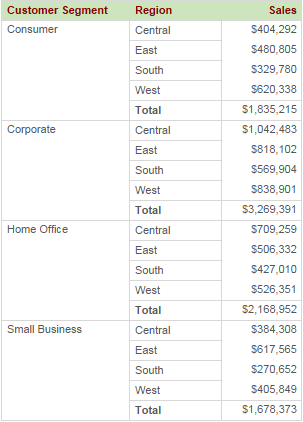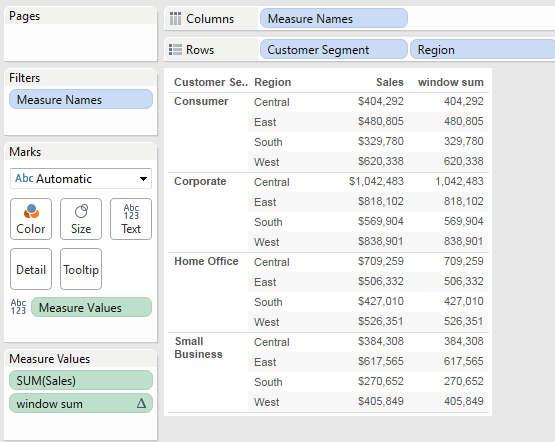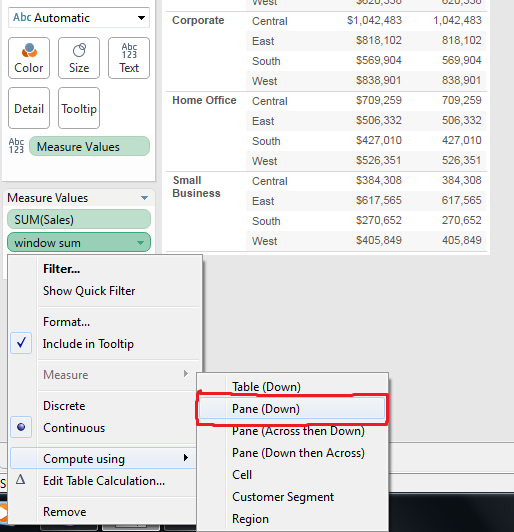# Tableau - Real Time Scenario 6 (Showing Sum(sales) for pane without repeating same value)

Problem: Showing Sum(sales) for pane with out repeating same value.
See below:In above image I am showing sum(Sales) for each customer segment by each pane.

The above scenario we can show in two different ways. But User want to see like above only.

See the other two ways below:In this method we are showing subtotal for every customer segment.Here we are showingsum(sales) at customer segment level usingwindow_sum() per eachpane.

In above image we are seeing sum(Sales) values for each row. Same value is repeating for each row in the pane.
But user wants to see only one value per each paneHow to do it?

Solution:

Its very simple but since longtime I don’t know this.

Step 1: Create calculated field “window_sum” using below formula.

if last()=0 then window_sum(sum(Sales))
else
NULL
END

Step 2: Drag Customer SegmentRegionSales and window_sum to row shelf. Then go to show me and select first row first option. See belowThen it will create a text table like belowStep 3: Now right click on “window_sum” column which is there in measure values shelf and go to “Compute using” then select “Pane Down”. See belowStep 4: Now you will see “Window_sum along pane (Down)” column header in the report. Just right click on that and select edit alias. Just give “sum(Sales)

Finally report look like below: